# Atoms

• Most of the Universe consists of matter and energy. Energy is the capacity to do work. Matter has mass and occupies space.
• All matter is composed of basic elements that cannot be broken down to substances with different chemical or physical properties.
•  Elements are substances consisting of one type of atom, for example Carbon atoms make up diamond, and also graphite.
• Pure (24K) gold is composed of only one type of atom, gold atoms. Atoms are the smallest particle into which an element can be divided.
• The ancient Greek philosophers developed the concept of the atom, although they considered it the fundamental particle that could not be broken down.
• Since the work of Enrico Fermi and his colleagues, we now know that the atom is divisible, often releasing tremendous energies as in nuclear explosions or (in a controlled fashion in) thermonuclear power plants.

Subatomic particles were discovered during the 1800s. For our purposes we will concentrate only on three of them, summarized in Table 1. The proton is located in the center (or nucleus) of an atom, each atom has at least one proton. Protons have a charge of +1, and a mass of approximately 1 atomic mass unit (amu). Elements differ from each other in the number of protons they have, e.g. Hydrogen has 1 proton; Helium has 2.

SUBATOMIC PARTICLES STRUCTURE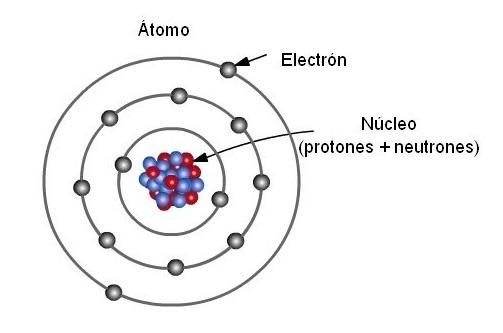The neutron also is located in the atomic nucleus (except in Hydrogen). The neutron has no charge, and a mass of slightly over 1 amu. Some scientists propose the neutron is made up of a proton and electron-like particle.

The electron is a very small particle located outside the nucleus. Because they move at speeds near the speed of light the precise location of electrons is hard to pin down.

Electrons occupy orbitals, or areas where they have a high statistical probability of occurring. The charge on an electron is -1. Its mass is negligible (approximately 1800 electrons are needed to equal the mass of one proton).

Table 1. Subatomic particles of use in biology.

 Name Charge Location Mass Proton +1 atomic nucleus 1.6726 X 10-27 kg Neutron 0 atomic nucleus 1.6750 X 10-27 kg Electron -1 electron orbital 9.1095 X 10-31 kg

The atomic number is the number of protons an atom has. It is characteristic and unique for each element. The atomic mass (also referred to as the atomic weight) is the number of protons and neutrons in an atom.

• Atoms of an element that have differing numbers of neutrons (but a constant atomic number) are termed isotopes.
• Isotopes, shown in Figure 1 and Figure 2, can be used to determine the diet of ancient peoples by determining proportions of isotopes in mummified or fossilized human tissues.
• Biochemical pathways can be deciphered by using isotopic tracers.
• The age of fossils and artifacts can be determined by using radioactive isotopes, either directly on the fossil (if it is young enough) or on the rocks that surround the fossil (for older fossils like dinosaurs).
• Isotopes are also the source of radiation used in medical diagnostic and treatment procedures.

Figure 1. Note that each of these isotopes of hydrogen has only one proton. Isotopes differ from each other in the number of neutrons, not in the number of protons.

ISOTOPES OF HYDROGEN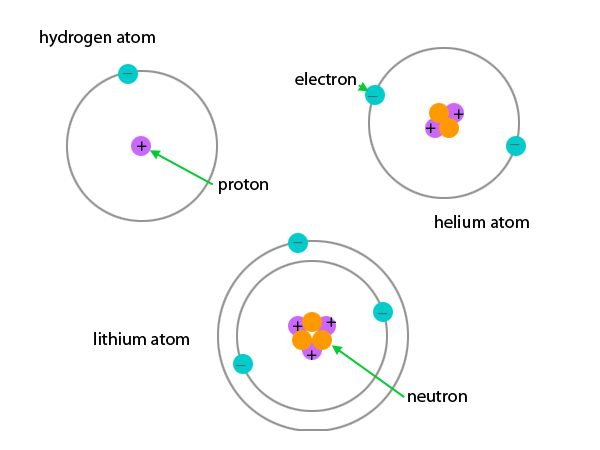Some isotopes are radioisotopes, which spontaneously decay, releasing radioactivity. Other isotopes are stable. Examples of radioisotopes are Carbon-14 (symbol 14C), and deuterium (also known as Hydrogen-2; 2H). Stable isotopes are 12C and 1H.

Figure 2. Carbon has three isotopes, of which carbon-12 and carbon-14 are the most well known. Image from Purves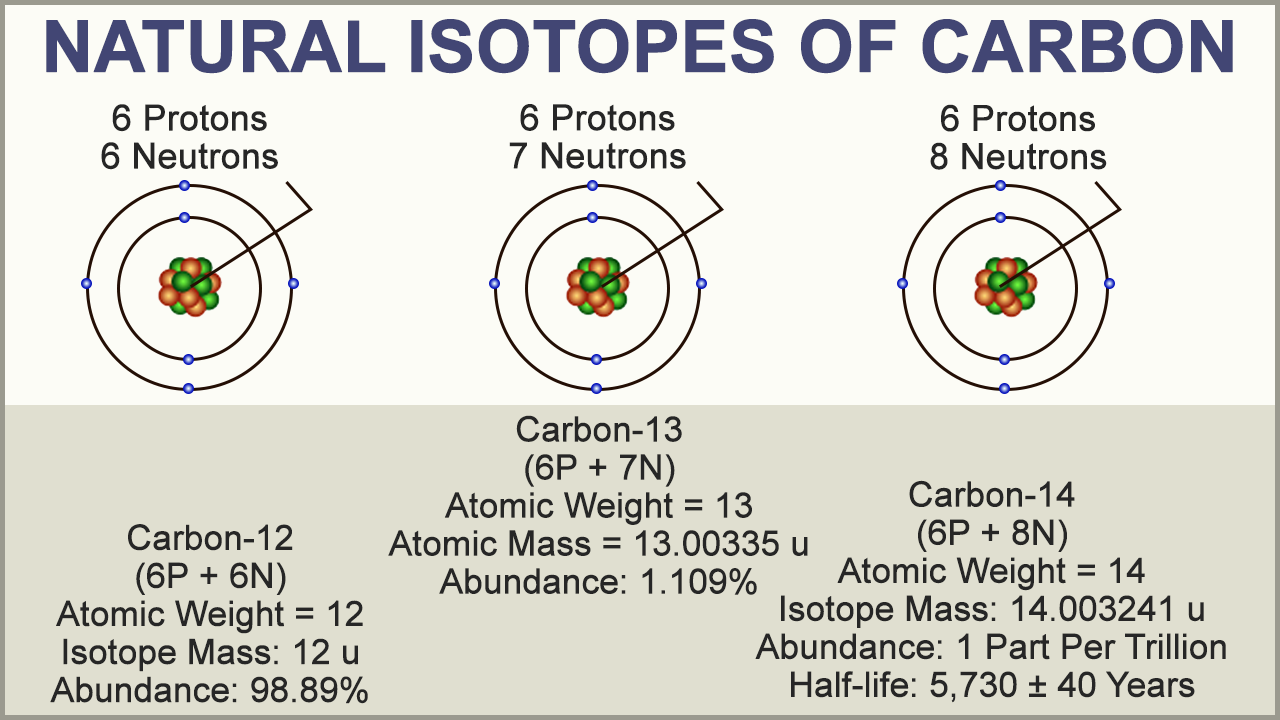The Periodic Table of the Elements, a version of which is shown in Figure 3, provides a great deal of information about various elements.

 Figure 3. The Periodic Table of the Elements. Each Roman numeraled column on the label (at least the ones ending in A) tells us how many electrons are in the outer shell of the atom. Each numbered row on the table tells us how many electron shells an atom has. Thus, Hydrogen, in column IA, row 1 has one electron in one shell. Phosphorous in column VA, row 3 has 5 electrons in its outer shell, and has three shells in total. Image from James K. Hardy’s chemistry site at the University of Akron.periodic table explained in the video below

# Compounds, Formulae and Equations

Key Facts & Summary

• Elements are composed of one type of atom. An element cannot be decomposed into simple substances
• Compounds can be decomposed into elements
• Compounds are composed of two or more types of atoms
• A Chemical formula is a representation of the number of atoms of each element that makes a compound.
• The different types of formulas are molecular, empirical, structure, and condensed chemical formulas.
• A chemical equation represents the processes that occur during a chemical reaction, using numbers and symbols
• A chemical reaction needs to be balanced.

Compounds

When two or more elements are chemically joined, a compound is formed. Water, salt, and sugar are examples of compounds. When the elements are joined, the atoms lose their individual properties and acquire different properties.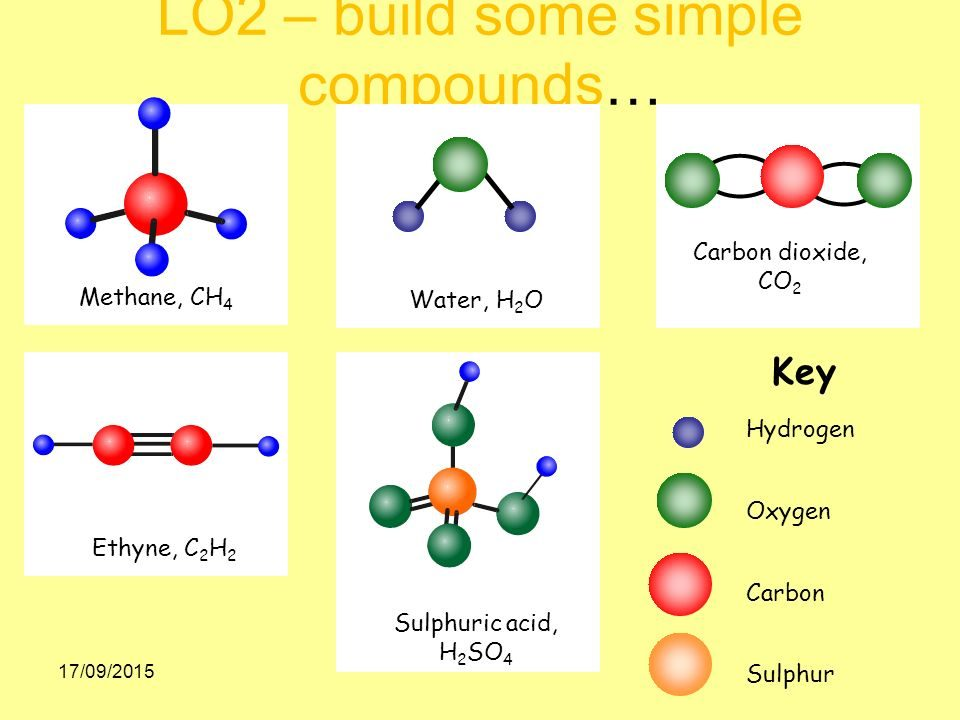Some compounds are made of molecules (linked collection of atoms like H2O) and some are made of ions (positive and negative charged atoms) NaCl  is made of a number Na+ and Cl ions in a three-dimensional array but NOT NaCl molecules.

A chemical formula is used a quick way to show the composition of compounds. Letters, numbers, and symbols are used to represent elements and the number of elements in each compound.

## Chemical formulae

• Each substance present in nature has a specific chemical composition, and therefore they have their own unique chemical formula.
• Simply put, a chemical formula is a representation of the number of atoms of each element that makes a compound. It contains the symbols of the atoms of the elements present in the compound and the number of each element in the form of subscripts.
• For example in the glucose, the sugar you use to sweetener the coffee there are 12 carbon (C) atoms, 22 hydrogen (H) atoms and 11 oxygen (O) atom, so the chemical formula is C6H12O6
• Chemical formulas can be quite simple such as the formula for hydrogen, which is H, or it can be much more complicated, such as the formula for ethanol, a particular alcohol, CH3CH2OH.
• Also, there are different types of formulas, including molecular, empirical, structure, and condensed chemical formulas.

## Molecular Formula

• Also known as the “true formula”, the molecular formula states the actual number of atoms of the elements in a single molecule. For example, as we have seen above, the molecular formula of the sugar glucose is C6H12O6.

## Empirical Formula

• The empirical formula gets its name from empirical data and it represents the simplest ratio of the whole number of elements in a compound. It can be the same as the molecular formula, as it happens with the formula of water (H2O), while other times the formulae are different. For example, the empirical formula of glucose is CH2O, which is obtained by dividing all of the subscripts by the common value (6, in this case).

## Structural Formula

• A structural formula shows the chemical bonds, and how the atoms are arranged.
• This is important information because two molecules may have the same number and type of atoms, but arranged in a different way. (Figure 1)

For example, ethanol (common alcohol people can drink) and dimethyl ether (a toxic compound) share the same molecular and empirical formulas but have a different structural formula. This means that the same atoms are arranged in a different way.

structural formulae of ethanol and dimethyl ether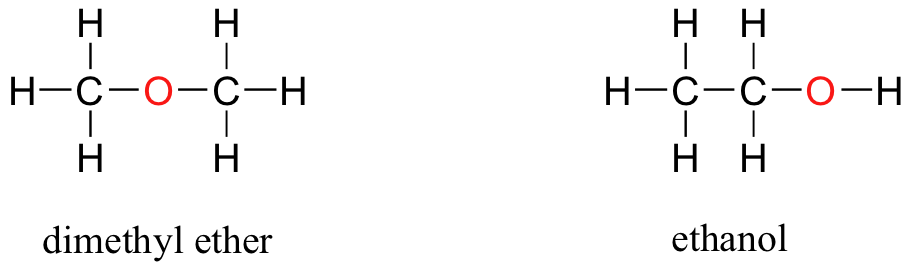Condensed Structural Formula

The condensed structural formula may omit the symbols for carbon and hydrogen in the structure, simply indicating the chemical bonds and formulas of functional groups. It is important because this formula indicates the position of the atoms and the functional group in the molecule structure, which is quite useful when studying organic chemistry. The written condensed formula lists the atoms in the order in which they appear in the molecular structure. For example, the molecular formula of hexane is C6H14, but its condensed formula is CH3(CH2)4CH3.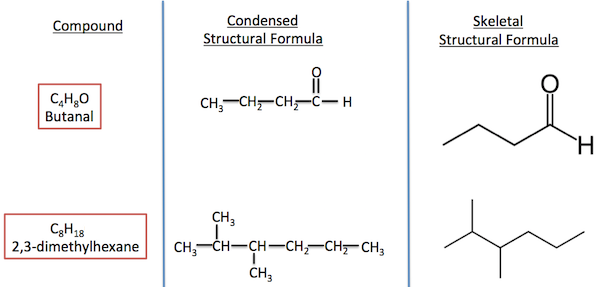Chemical equations

A chemical equation is a way to represent the processes that occur during a chemical reaction, using numbers and symbols.

The reactants of the reactions are written on the left side of an arrow, and the products are written on the right side. The arrow usually points to the products, but on some occasions is directed in both way, indicating an equilibrium, where the chemical reaction is proceeding in both way, at the same time.

The elements in an equation are denoted using their symbols. Coefficients next to the symbols indicate the stoichiometric numbers. Subscripts are used to indicate the number of atoms of an element present in a chemical species.

Methane + Oxygen →  Carbon Dioxide  + Water

CH4 + 2O2  →  CO2  + H2O

The participants in the chemical reactions are element symbols. In this reaction, C is carbon, H is hydrogen, and O is oxygen. Usually, you will need to know the element symbols, in order to understand the equations.

The reactants  are written on the left side of the arrow, and in this  reaction are CH4 + O2

The products are written on the right side of the arrow, and in this  reaction are  CO2  + H2O

The arrow between the reactants and products should point from left to right or, if the reaction is proceeding both ways, point in both directions (this is common). If your arrow points from right to left, it’s a good idea to re-write the equation the conventional way.

Balancing Mass and Charge

Chemical reactions need to be balanced.  An unbalanced equation lists the reactants and products, but not the ratio between them. A balanced chemical equation has the same number and types of atoms on both sides of the arrow. If ions are present, the sum of the positive and negative charges on both sides of the arrow is also the same.

Dissolve table salt is a chemical change :

NaCl    Na+ + Cl

ions form and spread out in water. Note that the sum of the charges present on the left and on the right of the arrow, is the same: this reaction is chemically balanced.

Indicating States of Matter

It’s common to indicate the state of matter in a chemical equation by including parentheses and an abbreviation right after a chemical formula. Gases are indicated by (g), liquid by (l). Aqueous solution are indicated by (aq) which means that the chemical species is in water as an aqueous solution.
UNDERSTANDING COMPOUNDS, FORMULEA AND EQUATIONS

# IONS

An ion is an atom with more or less electrons than protons and therefore has a charge – either positive or negative.

If it has more electrons than protons it has a net negative charge and is known as an anion.

If it has fewer electrons than protons it has a net positive charge and is known as a cation.

The ionic state may be denoted as a following superscript (e.g. O2-, Fe2+). The number in front of the charge symbol shows how many electrons the atom has gained or lost. O2- is an oxygen atom that has gained 2 electrons.

The common ionic states of an atom are known as its valences.

Name and Formulae of Common Ions

Some Common Ions

 Cations Anions Charge Formula Name Charge Formula Name 1+ Na+ sodium ion 1- H– hydride ion K+ potassium ion Cl– chloride ion Cu+ copper(I) ion Br– bromide ion Ag+ silver ion I– iodide ion Hg+ mercury(I) ion OH– hydroxide ion H+ hydrogen ion NO3– nitrate ion NH4+ ammonia ion NO2– nitrite ion HCO3– hydrogencarbonate HSO4– hydrogensulphate CN– cyanide MnO4– permengante ClO3– chlorate ClO– chlorite 2+ Mg2+ magnesium ion 2- O2- oxide Ca2+ calcium ion S2- sulpide Ba2+ barium ion SO42- sulphate Pb2+ lead(II) ion SO32- sulphite Fe2+ iron(II) ion S2O32- thiosulphate Co2+ cobalt(II) ion SiO32- silicate Ni2+ nickel(II) ion CO32- carbonate Mn2+ manganese(II) ion CrO42- chromate Cu2+ copper(II) ion Cr2O72- dichromate Zn2+ zinc ion Hg2+ mercury(II) ion 3+ Al3+ aluminium ion 3- N3- nitride ion Fe3+ iron(III) ion P3- phosphide ion Cr3+ chromium ion PO43- phosphate ion

Ionic bonding and advanced electron notation

I’m not going to repeat the diagrams, there’s no point, so I’m just outlining the ionic bond formation in s, p and d terms.

Most involve the ‘octet rule’ to form a noble gas electron configuration.

Examples

You need to be able to write the electron configuration of ions in terms of s, p and d orbital notation.

e.g. sodium ion Na+ is 1s22s2p6, [Ne], and the chloride ion Cl is 1s22s2p63s23p6, [Ar]

Which elements form ionic compounds? and how to work out and write an ionic formula?

 Pd metals Part of the modern Periodic Tablerelated to selected elements that form ionic compounds Pd = period, Gp = group metals => non–metals Gp1 Gp2 Gp3 Gp4 Gp5 Gp6 Gp7 Gp0 1 1H  Note that H does not readily fit into any group 2He 2 3Li 4Be atomic number Chemical Symbol eg 4Be 5B 6C 7N 8O 9F 10Ne 3 11Na 12Mg 13Al 14Si 15P 16S 17Cl 18Ar 4 19K 20Ca 21Sc 22Ti 23V 24Cr 25Mn 26Fe 27Co 28Ni 29Cu 30Zn 31Ga 32Ge 33As 34Se 35Br 36Kr 5 37Rb 38Sr 39Y 40Zr 41Nb 42Mo 43Tc 44Ru 45Rh 46Pd 47Ag 48Cd 49In 50Sn 51Sb 52Te 53I 54Xe 6 55Cs 56Ba Transition Metals 81Tl 82Pb 83Bi 84Po 85At 86Rn Gp 1 Alkali Metals  Gp 2 Alkaline Earth Metals  Gp 7/17 Halogens  Gp 0/18 Noble GasesChemical bonding comments about the selected elements highlighted in white e.g. When the electropositive metals on the left combine with the non–metals on the right, quite often ionic bond is formed e.g. the formation of an ionic compound like sodium chloride NaCl Note: Throughout this page to form stable ions with a noble gas electron arrangement by electron transfer … (a) Group 1 metals lose their 1 outer electron to form a singly charged positive ion: M ==> M+ + e– (b) Group 2 metals lose their 2 outer electrons to form a doubly charged positive ion: M ==> M2+ + 2e– (c) Group 6 non–metals gain 2 electrons to form a doubly charged negative ion: X + 2e– ==> X2– (d) Group 7 halogen non–metals gain 1 electron to form a singly charged negative ion: X + e– ==> X– In a correct ionic formula: total positive ion charge = total negative ion charge therefore we can predict the following formula where  M = a group 1/2 metal  and  X = a group 6/7 non–metal: (a) Group 1  +  (c) Group 6  ===>  M2X  or  (M+)2X2–   (eg group 1 oxides or sulfides) (a) Group 1  + (d) Group 7  ===>  MX  or  M+X–   (eg group 1 halides) (b) Group 2  +  (c) Group 6  ===>  MX  or  M2+X2–   (eg group 2 oxides or sulfides) (b) Group 2  +  (d) Group 7  ===>  MX2  or  M2+(X–)2   (eg group 2 halides) Atoms of groups 4/14 and 5/15 may also form ions in combination with very electropositive metals examples – showing the formation of a noble gas structure Group 4/14: carbon forms ionic carbides: C (2.4) + 4e– ==> C4– (carbide ion, 2.8) examples: sodium carbide Na4C, magnesium carbide Mg2C Group 5/15: nitrogen forms ionic nitrides: N (2.5) + 3– ==> N3– (nitride ion, 2.8) Group 5/15: phosphorus forms ionic phosphides: P (2.8.5) + 3– ==> P3– (nitride ion, 2.8) examples: potassium nitride K3N, magnesium nitride Mg2N3, sodium phosphide Na3P formulae derived from Na+, K+, Mg2+ (see next section on working out ionic formulae) Note that the 3d block – transition metals also form many simple ionic compounds with the more electronegative non–metals.

Examples of how to work out an ionic formula

numerical ion charges = the valency of A and B to deduce the formula AxBy

i.e. the valence or ionic charge = the combining power of the ion

‘molecular’ or ionic style of formula and compound name are shown

In the electrically balanced formula for a potentially stable compound, the total positive ionic charge must equal the total negative ionic charge.

number of positive ion ‘A’ x positive charge of ion ‘A’ = number of negative ion ‘B’ x negative charge of ion ‘B’ (you ignore charge sign)

Example: A moderately difficult example to work out!

Aluminium oxide consists of aluminium ions Al3+ and oxide ions O2–

number of Al3+ x charge on Al3+ = number of  O2– x charge on O2–

the simplest whole number ratios are 2 of Al3+ x 3 = 3 of  O2– x 2 (total 6+ balances total 6–)

so the simplest whole number formula for aluminium oxide is Al2O3 which is its empirical formula

More examples of how to work out ionic formulae

1 of Kbalances 1 of Br because 1 x 1 = 1 x 1 gives KBr or K+Br    potassium bromide

2 of Nabalances 1 of O2– because 2 x 1 = 1 x 2 gives Na2O or (Na+)2O2–    sodium oxide

1 of Mg2+ balances 2 of Cl because 1 x 2 = 2 x 1 gives MgCl2 or Mg2+(Cl)2    magnesium chloride

1 of Fe3+ balances 3 of F because 1 x 3 = 3 x 1 gives FeF3 or   Fe3+(F)3    iron(III) fluoride

1 of Ca2+ balances 2 of NO3 because 1 x 2 = 2 x 1 gives Ca(NO3)2 or Ca2+(NO3)2     calcium nitrate

2 of Fe3+ balances 3 of SO42– because 2 x 3 = 3 x 2 gives Fe2(SO4)3 or (Fe3+)2(SO42–)3    iron(III) sulfate

(includes common oxides, hydroxides, carbonates, hydrogencarbonates, halides, sulfates, nitrates)

At Advanced A level you would be expected to work out any ionic formulae from given ions and you should know many of them anyway, or work out the charge on the ion from the position of the element in the periodic table and using the octet electron rule eg for groups 1, 2, 3/13, 4/14, 5/15, 6/16, 7/17, 0/18.

Naming ionic inorganic compounds

When combined with other elements in simple compounds the name of the non-metallic element changes slightly from …??? to …ide.

Sulfur forms a sulfide (ion S2-), oxygen forms an oxide (ion O2-), fluorine forms a fluoride (ion F), chlorine forms a chloride(ion Cl), bromine a bromide (ion Br) and iodine an iodide (ion I).

The other element at the start of the compound name e.g. hydrogen or a metal like sodium, potassium, magnesium, calcium, etc. remains unchanged because there is only one oxidation state.

So typical compound names are, sodium sulfide, hydrogen sulfide, magnesium oxide, potassium fluoride, hydrogen chloride, sodium chloride, calcium bromide, magnesium iodide etc.

However, with different oxidation states the complications will arise e.g.

(i) Where an element can form two different compounds with different formulae with the same  element there needs to be a way of expressing it in the name as well as in the formula e.g.

iron(II) chloride, FeCl2 and iron(III) chloride, FeCl3

copper(I) oxide, Cu2and copper(II) oxide, CuO

Hear chlorine has a combining power of 1 (valence 1) and oxygen 2 in both compounds.

However, iron can have a valence of 2 or 3 and copper 1 or 2 and these also correspond numerically to the charge on the metal ions in such compounds e.g. Fe2+ and Fe3+, Cu+ and Cu2+.

Therefore the ‘Roman numerals’ number in (brackets) gives the valence of the element in that particular compound. At a higher academic level this is known as the oxidation state.

(ii) When the non-metal is combined with oxygen to form a negative ion (anion) ion which combines with a positive ion (cation) from hydrogen or a metal, then the end of the 2nd part of the name ends in …ate or …ite e.g.

NO3 in a compound formula is nitrate e.g. KNO3, potassium nitrate.

SO3 in a formula is sulphite, e.g. Na2SO3, sodium sulphite,

SO4 is sulfate, e.g. MgSO4, magnesium sulfate,

PO4 is phosphate, e.g. Na2HPO4, disodium hydrogen phosphate

• Other examples

For metallic or non–metallic elements the name of the element is used if NOT in an anion

• Some of the old names are still in common use, but try to use the correct systematic name e.g.

• copper(I) oxide Cu2O and copper(II) oxide CuO

• (once called cuprous oxide and cupric oxide)

• iron(II) chloride FeCl2 and iron(III) chloride FeCl3

• (once called ferrous chloride and ferric chloride)

• iron(II) oxide FeO, iron(III) oxide Fe2O3 and diiron(II) iron(III) oxide, Fe3O4

• (once called ferrous oxide and ferric oxide and tri–iron tetroxide)

• Historic note: …ous was the lower oxidation state, …ic the higher.

• sulfur(IV) oxide SO2 (sulfur dioxide) and sulfur(VI) oxide, SO3 (sulfur trioxide)

• nitrogen(I) oxide N2O (dinitrogen oxide) nitrogen(II) oxide NO (nitrogen monoxide), nitrogen(IV) oxide NO2(nitrogen dioxide) and nitrogen(V) oxide N2O5 (nitrogen pentoxide).

• transition metal complex cations e.g.

• diaquatetraamminecopper(II) ion, [Cu(H2O)2(NH3)4]2+

• (water and ammonia are electrically neutral ligands attached to the central Cu2+ ion)

• For elements (metal or non–metal) combined with oxygen or other more electronegative element, giving an anion, the ion name ends in …ate with the prefix derived from the elements name. In such cases the oxygen carries the negative oxidation state of (–2) or chlorine (–1) e.g.

• manganate(VI) ion, MnO42–manganate(VII) ion, MnO4, (was called the permanganate ion)

• sulfate(IV) ion, SO32– (sulphite) and sulfate(VI) ion,SO42– (sulfate)

• nitrate(III), NO2 (nitrite) and nitrate(V), NO3 (nitrate)

• Historic note: ite was the lower oxidation state, ate the higher.

• chlorate(I), ClOchlorate(VII), ClO4 etc. oxygen is more electronegative than chlorine.

• (once called the hypochlorite ion and the perchlorate ion respectively)

• transition metal complex anions e.g.

• tetrachlorocuprate(II) ion, [CuCl4]2– (oxidation states Cu +2, Cl –1)

## STEM Elearning

We at FAWE have built this platform to aid learners, trainers and mentors get practical help with content, an interactive platform and tools to power their teaching and learning of STEM subjects, more